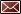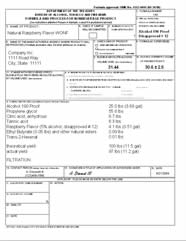Get Email Updates Back to Nonbeverage Products Laboratory page

# Drawback Tutorial

Example formulas

Filtration (includes a disapproved intermediate formula)

Note: disapproved formulas must be manufactured in-house

This is basically the same formula as in Simple mixture 2 except the Raspberry Essence has been changed to a disapproved formula and the product is filtered.  The ethanol from disapproved formulas is eligible for a drawback claim if it is used in an approved formula as an intermediate (it must be claimed in item 2 of TTB Form 5154.1).  In this case, both the Raspberry Essence and the 190 proof ethanol are eligible for drawback and will be used to calculate item 9.Step 1. Item 9 calculation – First source of eligible alcohol - calculate the absolute ethanol coming from the 190 proof ethanol 25.0 pounds ÷ 6.7943 pounds/gallon = 3.68 gallons multiply by the % ethanol 3.68 gallons × 0.95 = 3.50 gallons of absolute ethanol Second source of eligible alcohol - calculate the gallons of absolute ethanol from the disapproved Raspberry Essence by multiplying the volume by the % ethanol 0.51 gallons × 0.050 = 0.026 gallons of absolute ethanol Add the gallons of absolute alcohol together and divide by the actual yield (the actual yield is the amount of product left after processing… in this case, filtration) Click on the image to enlarge

(3.50 gallons of absolute ethanol + 0.026 gallons of absolute ethanol) ÷ 11.2 gallons = 0.315 or 31.5%

Step 2. Item 10 calculation –

In this example, the absolute gallons of ethanol are calculated the same as for item 9

3.50 gallons of absolute ethanol + 0.026 gallons of absolute ethanol = 3.526 absolute gallons of ethanol

divide this by the theoretical yield to get the ethanol content for item 10:

3.526 gallons of absolute ethanol ÷ 11.5 gallons = 0.307 or 30.7%

Since this is a filtered product one would use the tolerance established for filtrations in the tolerance table.